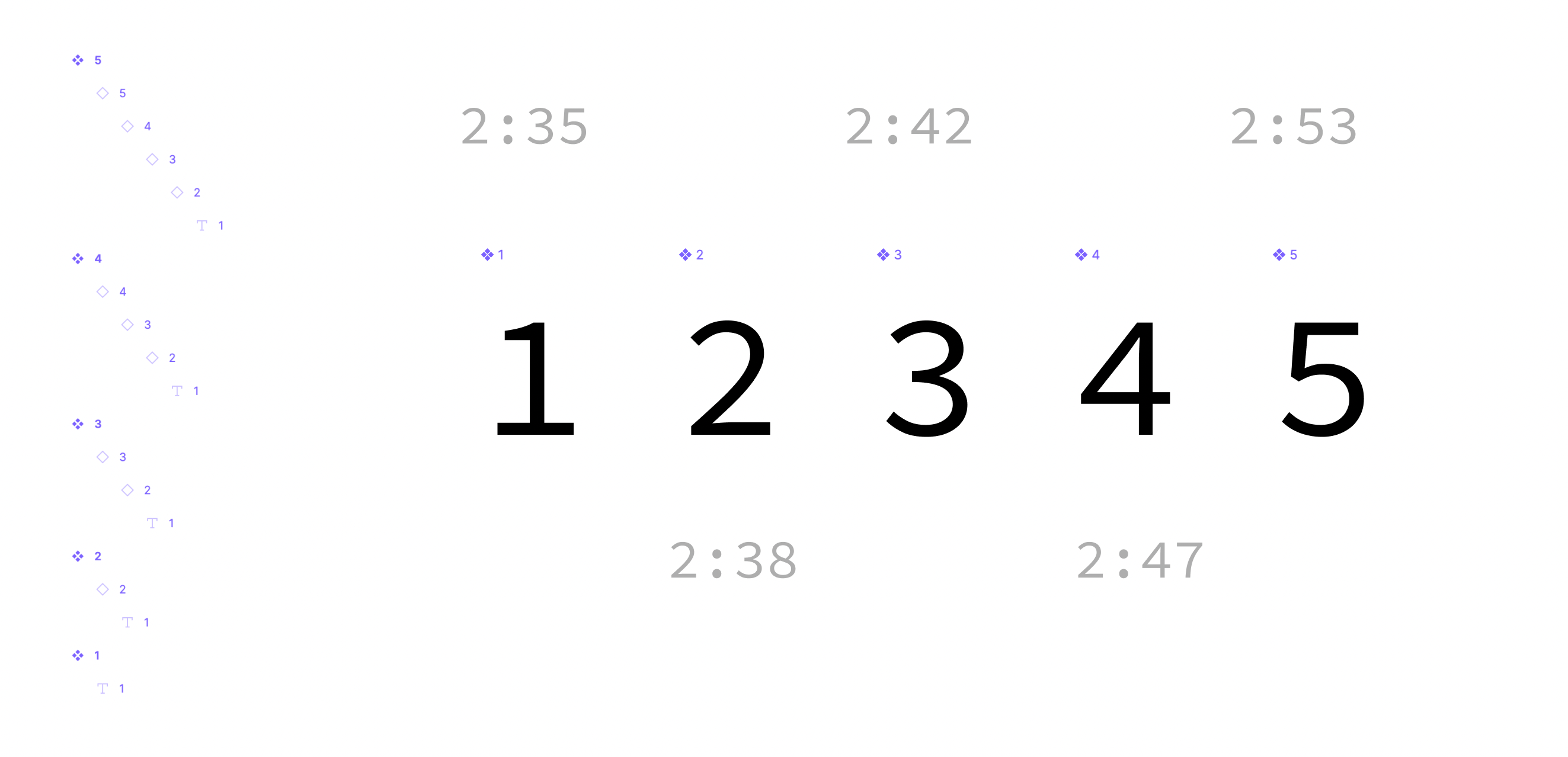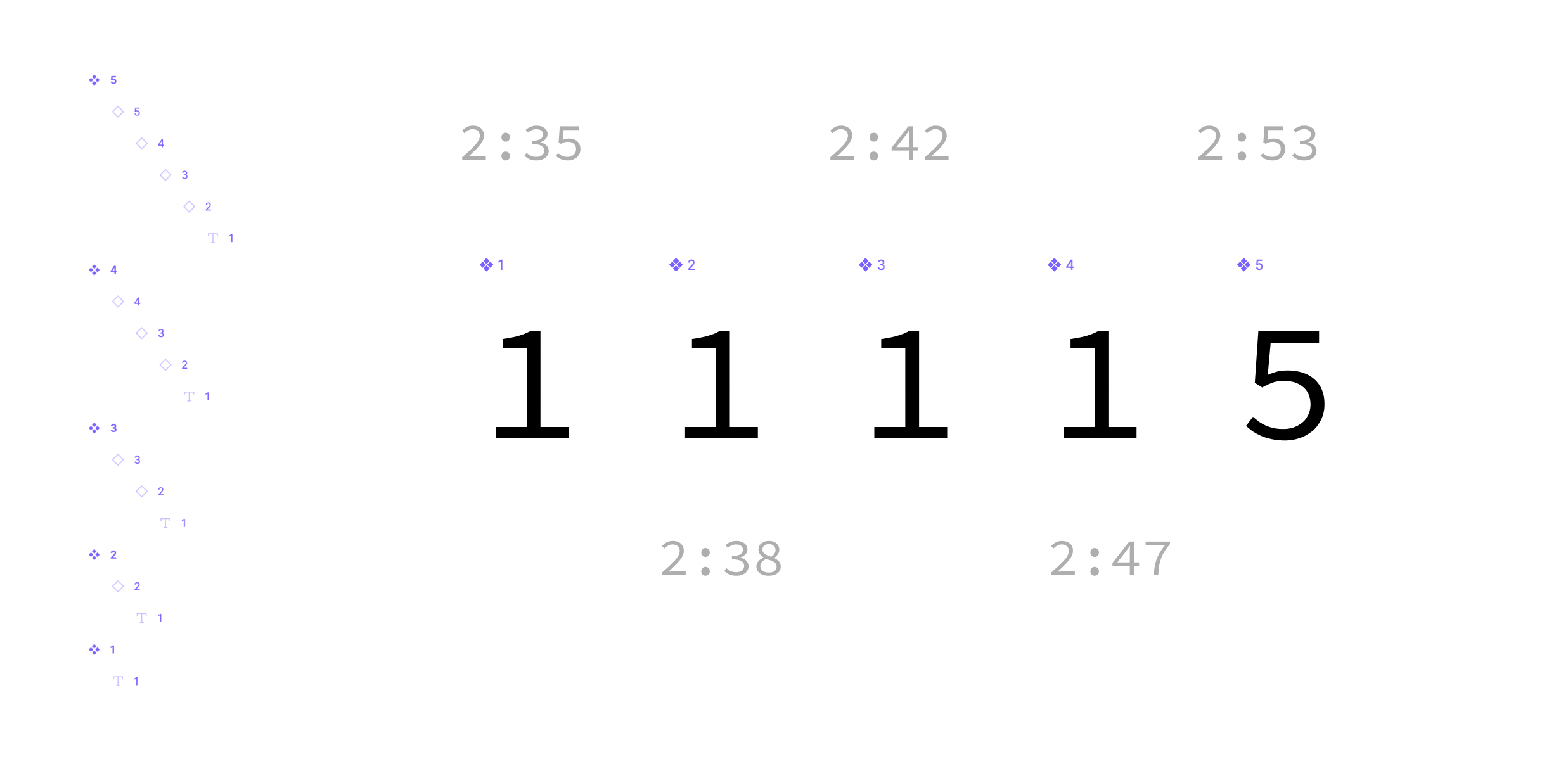# Understanding Nested ComponentsSo, let's define the scenario based on above image.

We have component 1 to 5, each instanciating a previous component. (except 1)

As the final tree structire is:

``- 1 master  - text- 2 master  - 1 instance instnace of 1    - text- 3 master  - 2 instance instance of 2    - 1 instance instance of 1      - text- 4 master  - 3 instance instance of 3    - 2 instance instance of 2      - 1 instance instance of 1        - text- 5 master  - 4 instance of 4    - 3 instance instance of 3      - 2 instance instance of 2        - 1 instance instance of 1          - text``

If you had only `1`, we'de define the component as:

``function _1() {  return <p>1</p>;}``

if with instanciation, with override text:

``function _1({ text = "1" }: { text: string }) {  return <p>{text}</p>;}``

If we had only `1` & `2` master components and `1'` & `2'` instanciation We'de define `1` & `2` as below

``function _1({ text = "1" }: { text: string }) {  return <p>{text}</p>;}function _2() {  return <_1 text="2" />;}``

The problem starts from 3rd. you might be thinking there's no big deal about this, but for computer iterating through the tree, it gets a bit tricky.

Since the `3` is instanciating `2`, but actually having the value overriden for `1` (which is instanciation of `2`).

``function _1({ text = "1" }: { text: string }) {  return <p>1</p>;}function _2() {  return <_1 text="2" />;}``

But what if case like this?If master `1` ~ `4` and `5` as a instance of `4`

``function _1() {  return <p>1</p>;}function _2() {  return <_1 />;}function _3() {  return <_2 />;}function _4() {  return <_3 />;}const _5 = <_4 />;``

If all `1` ~ `5` as a master

``function _1({ text = "1" }: { text: string }) {  return <p>{text}</p>;}function _2({ text = "2" }: { text: string }) {  return <_1 text={text} />;}function _3({ text = "3" }: { text: string }) {  return <_2 text={text} />;}function _4({ text = "4" }: { text: string }) {  return <_3 text={text} />;}function _5({ text = "1" }: { text: string }) {  return <_4 text="5" />;}``

And this will impact all the masters starting from `4` to the starting `1`.